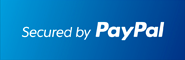** New: ECONOMICS , DIFFERENTIAL EQUATIONS **         ** SPECIALS for bulk apps **         ** Bestsellers: CALCULUS , PHYSICS , CHEM , STOCKS & PORTFOLIOS , ALGEBRA **How to get STEP BY STEP solutions using the TI89 Titanium Calculator

TI89 has a Computer Algebra System

You probably know that the TI 89 Titanium calculators are very powerful due to their built-in Computer Algebra System, called CAS.

This allows us to solve equations such as a*x + b = c for x or a or b or c. To accomplish this, just enter the following command into the HOME screen:

solve( a*x+ b = c, x) and press ENTER . Or type in solve( a*x+ b = c, b) when solving for b. The TI 83 / 84 calculators, however, cannot solve those

equations symbolically as they have no built-in CAS. For that reason, programs such as STEP BY STEP EQUATION SOLVER (www.Ti89.com)

can run on the TI 89 but not on the TI 83 / TI84 calculators. Interestingly, the TI 83 and TI 84 calculators are the most prominent calculators in the USA.

Prices between TI83/84 and the TI-89 Titanium calculators don't differ significantly. You could google : Graphing Calculator TI89 Walmart, or simply

Walmart calculators or Graphing Calculator TI 89 Amazon.

TI89 calculators excel in Calculus

May be the most important advantage of the TI 89 Titanium over the TI-83 and TI-84 calculators is in Calculus: Derivatives, Integrals and Limits can be

computed symbolically with ease using the TI-89 Titanium calculator. For example, integral(x^2,x,c) will correctly find x^3 / 3 + c . Or, if you like to have some fun you could

enter integral(g^2, g, p) to find g^3 / 3 + p . A TI-89 Titanium Derivative example would be d(x^3,x) = 3x^2 and d(x^3,x,2) finds the 2. derivative of x^3 .

The TI 89 is the best Limits Calculator, try this: Limit(sin(x)/x,x,0) to correctly get 1. If that does not convince you that the TI89 calculators

are the best Limits Calculator then enter this : limit((sin(x+h)-sin(x))/h,h,0) to correctly get its derivative cos(x) using the limit definition of the derivative.

Now that is powerful and it is the underlying reason why the TI 89 Titanium calculator app CALCULUS MADE EASY is such a powerful beast rivaling

WolframAlpha, Mathematica and Maple. This app takes full advantage of the CAS allowing for STEP by STEP solutions to both integrals and derivatives:

Just enter the function and press ENTER a few times to see all rules and steps involved! Considering you can carry the TI89 calculator with you, you practically

always have your personal tutor available, and that any time of day!

TI89 calculators excel in Differential Equations too

CALCULUS MADE EASY extends its STEP BY STEP solutions through Multivariable Calculus covering 4 years of Calculus. Thus, Step by Step Implicit

Differentiation, Step by Step Polar and Parametric Equations, Step by Step Partial Derivatives and Step by Step Lagrange Multipliers are all integrated. Even

Differential Equations can be solved Step by Step on the TI 89 Titanium using this app. Nevertheless, it is limited to the introductory method to solving Differential

Equations called Separation of Variables. In case you are looking for more advanced techniques such as Integration Factors, Variation of Parameter,

Undetermined Coefficients, Wronskian , Runge Kutta, LaPlace Transforms or you need to solve Higher Order, Bernoulli, Exact or Non-Exact or Linear Differerential

Equations then the DIFFERENTIAL EQUATIONS MADE EASY app will provide you with STEP BY STEP solutions on the TI89 Titanium calculator.

Step by Step solutions in Math, Science and Business

In fact, STEP by STEP apps for the TI89 Titanium calculator are available at www.TI89.com for all areas of Mathematics, Science and Business: Geometry,

Algebra , Trigonometry, PreCalculus , Statistics, Number Theory , Numerical and Complex Analysis, Physics, Chemistry, Business, Finances, even Portfolio & Markets.

Visit TI89.com to see the full listing of the 20+ STEP BY STEP apps available for the TI 89 Titanium Calculator. In fact, the Step by Step apps are also available for the

Voyage 200 calculator which also has the built-in CAS. The programmers at TI89.com take a lot of pride in their apps and gladly update them based on users feedback.

Trial versions are available and allow free testing of the apps.

TI89 old and new, RAM versus ROM

Lastly, we should mention that the TI89 Titanium is the modernized version of the original TI-89 calculator. While they both have CAS and are identical in functionality,

the newer TI89 Titanium calculator looks sleeker and has much more RAM as well as ROM. The TI89 Titanium calculator allows running more than 20 STEP BY STEP apps.

Apps are stored in ROM (the calculators hard drive) and are copied to RAM when executed and removed afterwards.